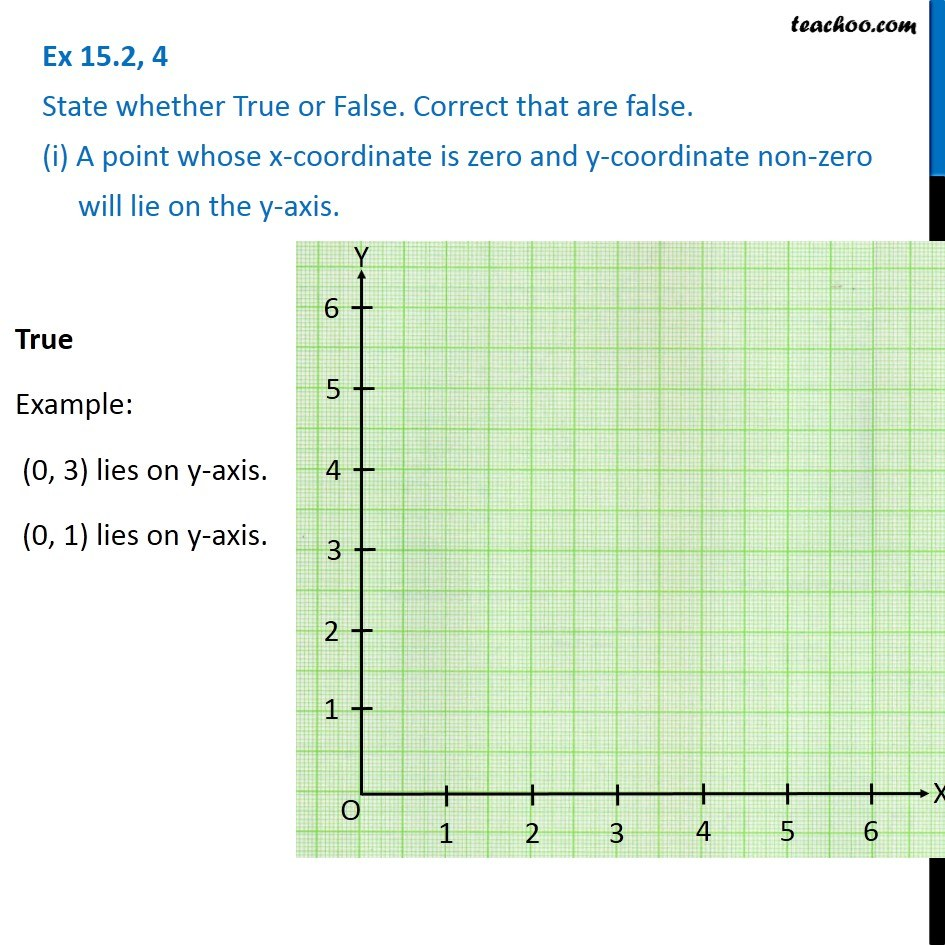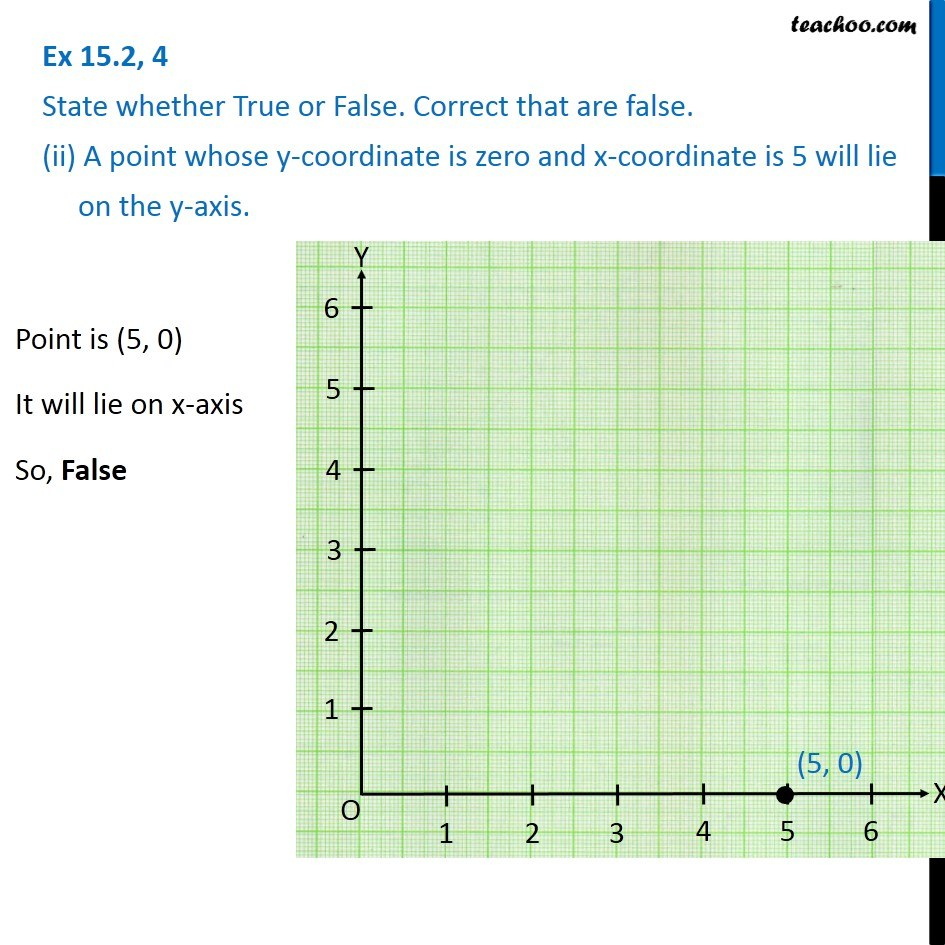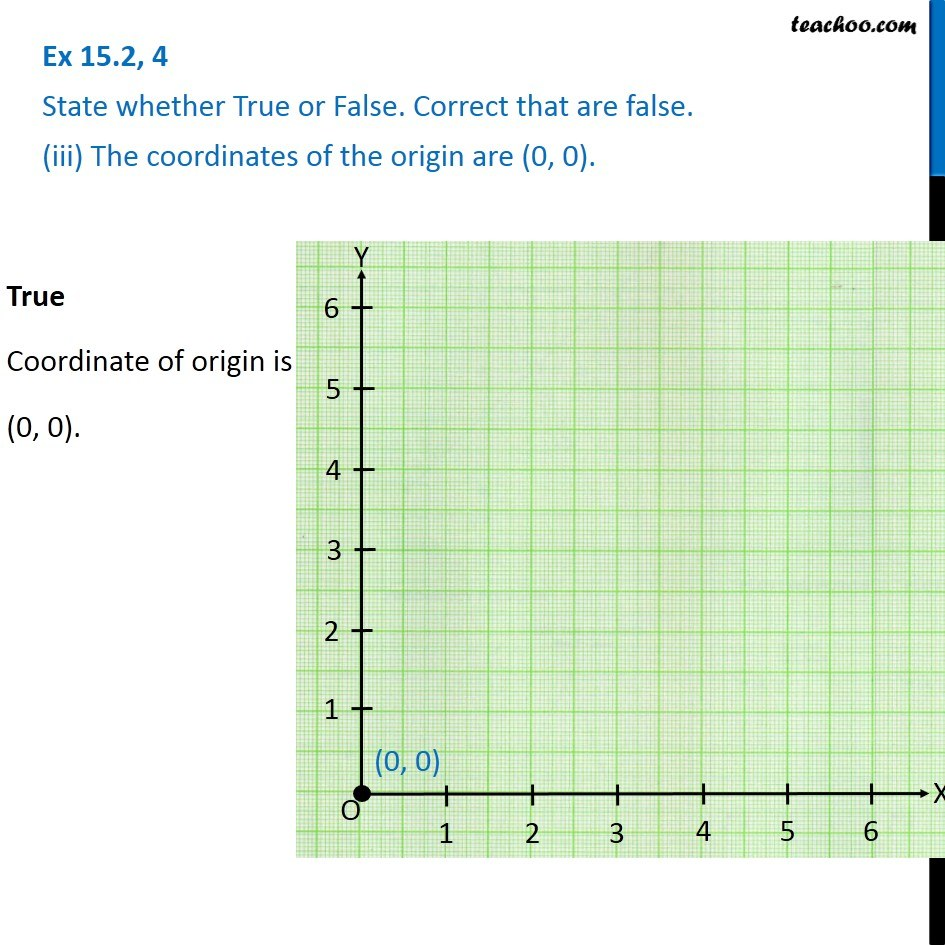1. Chapter 15 Class 8 Introduction to Graphs
2. Concept wise
3. Linear Graphs

Transcript

Ex 15.2, 4 State whether True or False. Correct that are false. (i) A point whose x-coordinate is zero and y-coordinate non-zero will lie on the y-axis. True Example: (0, 3) lies on y-axis. (0, 1) lies on y-axis. Ex 15.2, 4 State whether True or False. Correct that are false. (ii) A point whose y-coordinate is zero and x-coordinate is 5 will lie on the y-axis. Point is (5, 0) It will lie on x-axis So, False Ex 15.2, 4 State whether True or False. Correct that are false. (iii) The coordinates of the origin are (0, 0).True Coordinate of origin is (0, 0).

Linear Graphs